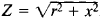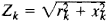# Electrical Impedance

(redirected from Phasor impedance)

## Electrical impedance

The measure of the opposition that an electrical circuit presents to the passage of a current when a voltage is applied. In quantitative terms, it is the complex ratio of the voltage to the current in an alternating current (ac) circuit.

A generalized ac circuit may be composed of the interconnection of various types of circuit elements. The impedance of the circuit is given by Z = V/I, where Z is a complex number given by Z = R + jX. R, the real part of the impedance, is the resistance of the circuit, and X, the imaginary part of the impedance, is the reactance of the circuit. The units of impedance are ohms. See Electrical resistance, Reactance

McGraw-Hill Concise Encyclopedia of Physics. © 2002 by The McGraw-Hill Companies, Inc.
The following article is from The Great Soviet Encyclopedia (1979). It might be outdated or ideologically biased.

## Impedance, Electrical

a quantity characterizing the opposition presented by a circuit to an alternating current. Often called simply impedance, it is measured in ohms. In the case of a sinusoidal alternating current, the impedance is expressed by the ratio of the amplitude of the voltage applied to the circuit terminals and the amplitude of the current throught the circuit; here, the impedance is equal to, where r is the resistance and x is the reactance. In the case of a nonsinusoidal alternating current, the impedance is determined separately for each kth har monic component:.